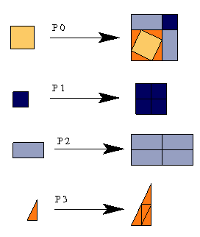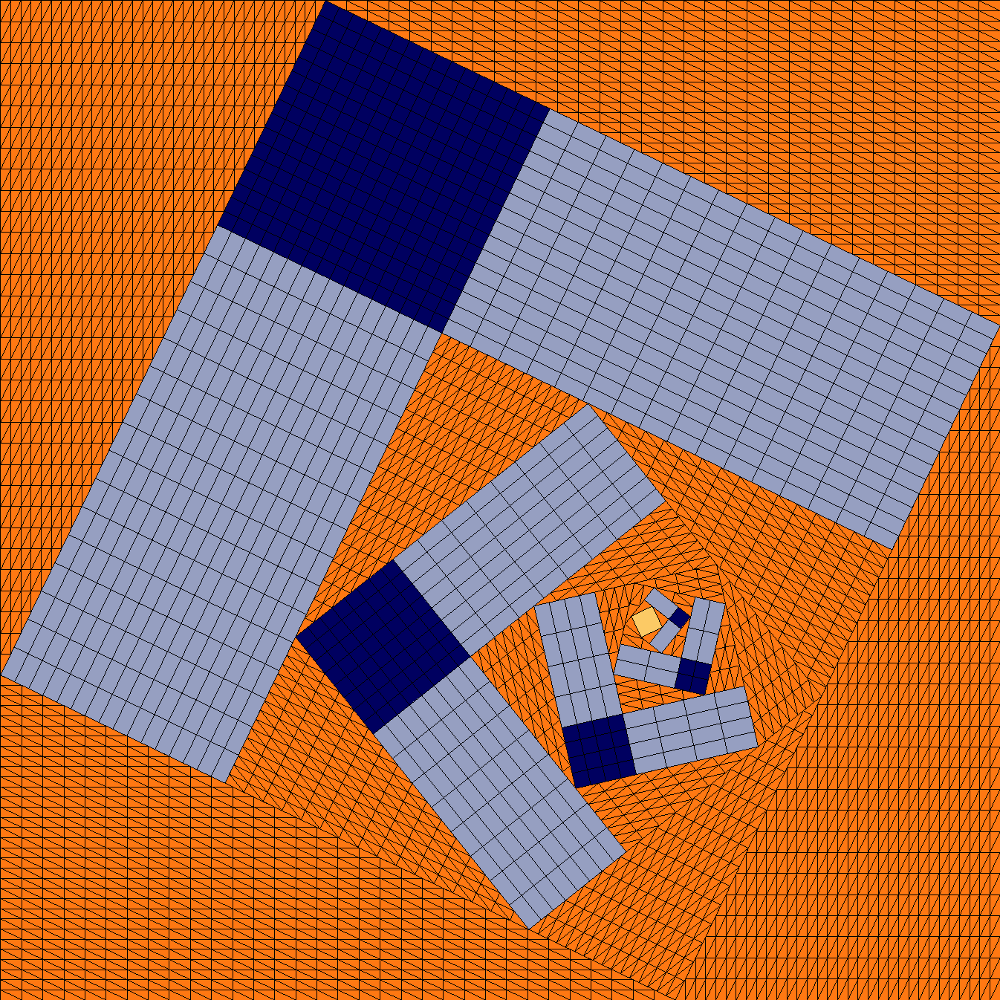## Cesi's Substitution

### Info

The substitution system uses 4 letters. With:

$x = \frac{\pi}{7}$, $c = \cos(x)$ and $s = \sin(x)$

They are: two squares of side lengths $1$ and $2-c-s$; a rectangle with sides $c+s$ and $2-c-s$: and a right triangle with legs $c$ and $s$. The substitution is indicated in the figure. Up to our knowledge, this was the first example of a substitution where the tiles occur in infinitely many orientations. Obviously, the substitution is not primitive.

Important: The substitution must be started with the large square to get anything interesting.

### Substitution Rule### Patch